Next: Input Impedance Up: Analysis Using Finite Open-Loop Previous: Analysis Using Finite Open-Loop

## Output Impedance

An op-amp will in general have a small resistive output impedance from the push-pull output stage. We will model the open-loop output impedance by adding a series resistor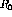to the output of an ideal op-amp as shown in figure 6.28.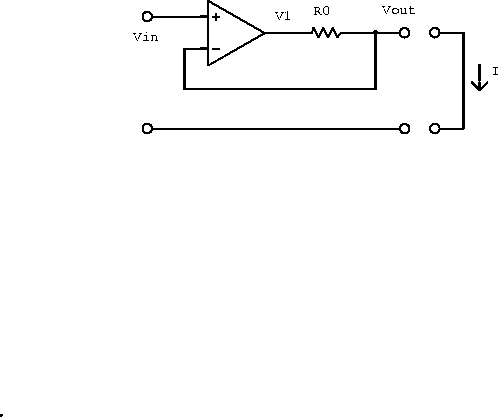Figure 6.28:  Real, current-limiting operational amplifier partially modeled by an ideal amplifier and an output resistor.

Assuming no current into the input terminals (unloaded), and hence no current through, we have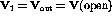. Using the open-loop transfer function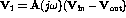we obtain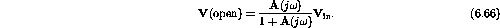Shorting a wire across the output gives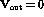and hence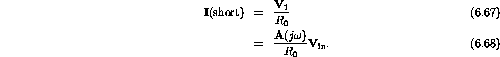Using the standard definition for the impedance gives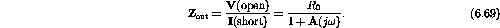If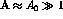than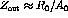, which is small as required by our infinite open-loop gain approximation.

We can now draw the impedance outside the feedback loop and use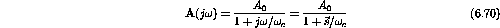to obtain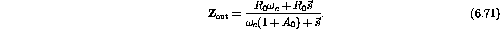The circuit can now be modeled by a resistor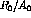in series with an inductor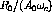all in parallel with another resistor(three passive components) as shown in figure 6.29. Students should convince themselves of this.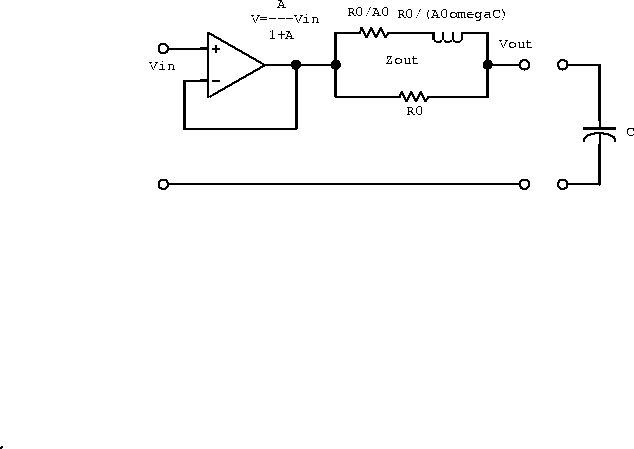Figure 6.29:  An equivalent circuit for a 741-type operational amplifier.

If the op-amp is used to drive a capacitive load, the inductive component in the output impedance could set up an LCR resonant circuit which would result in a slight peaking of the transfer function near the corner frequency as shown in figure 6.30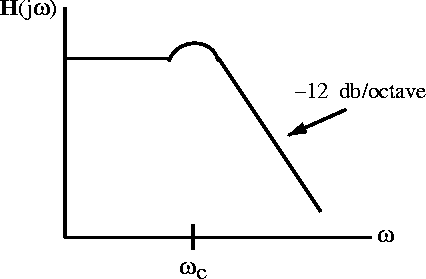Figure 6.30:  The overall transfer function when the amplifier drives a capacitive load.

Doug Gingrich
Tue Jul 13 16:55:15 EDT 1999